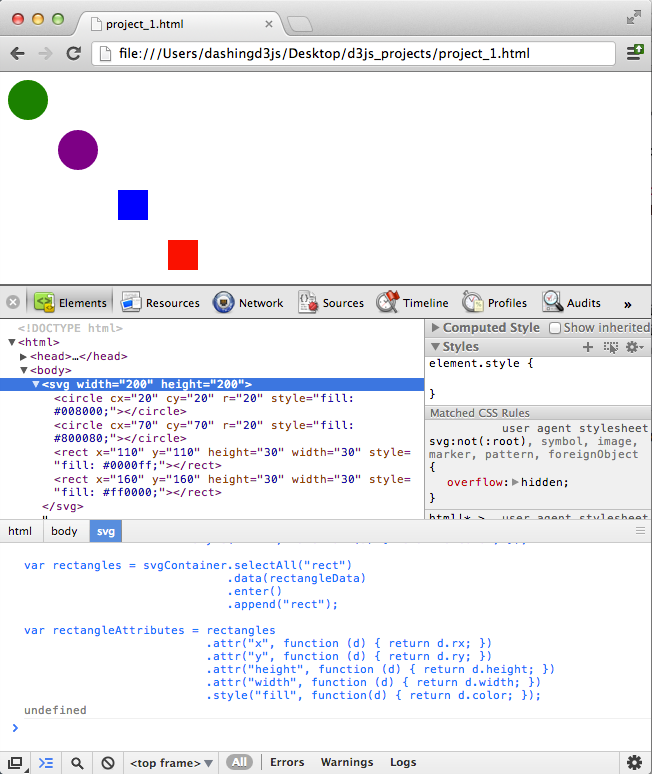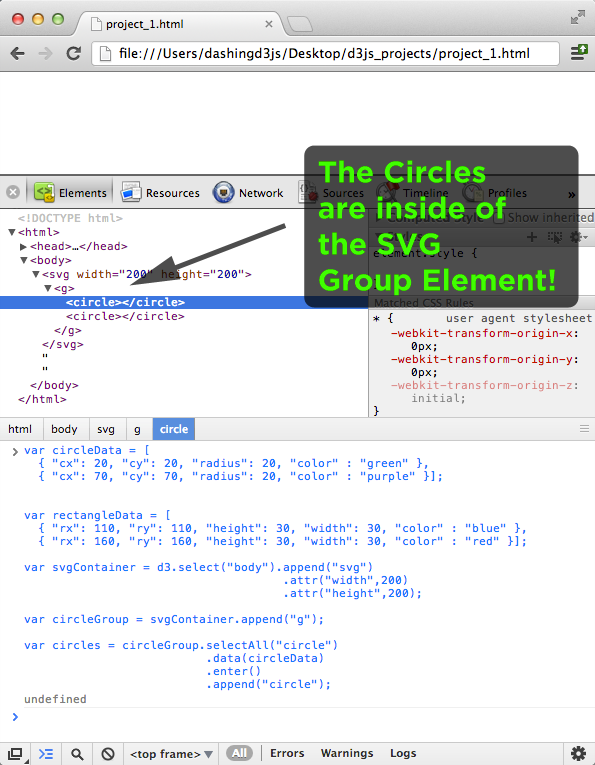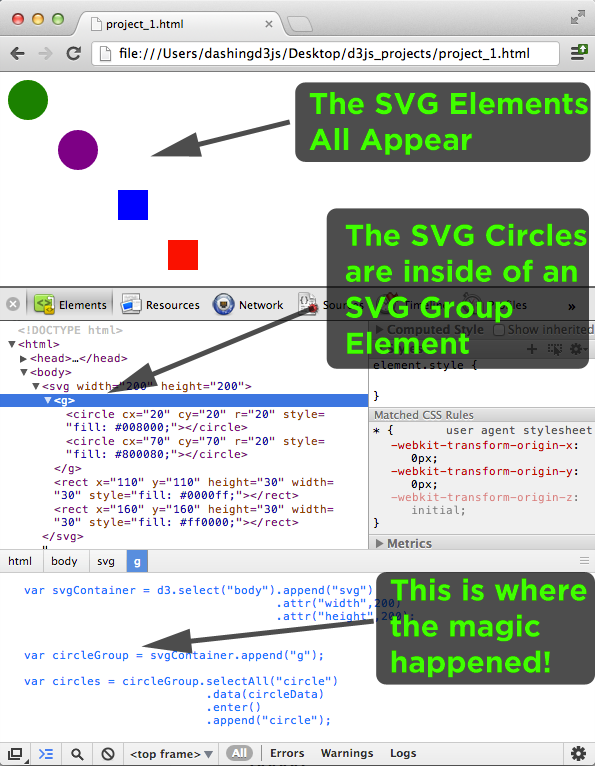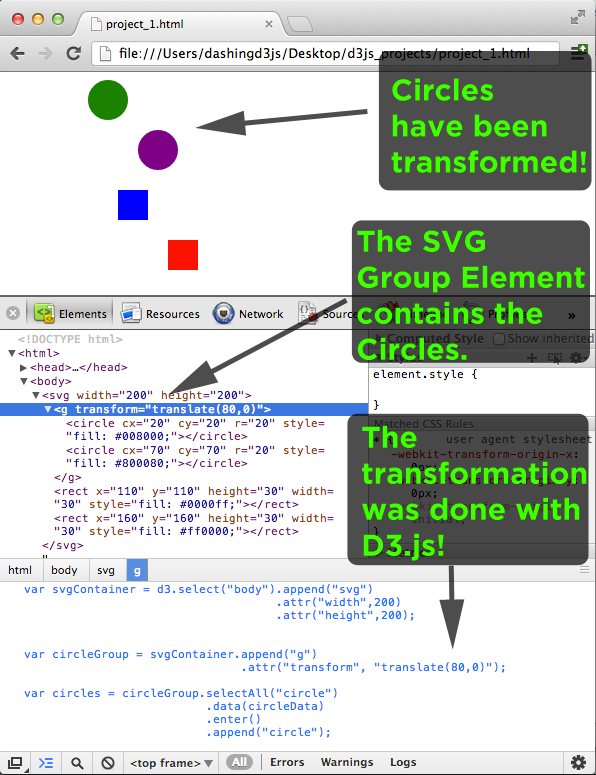# SVG Group Element and D3.js

### The Goal

In this section, we will cover the SVG Group Element, why it is important and how we use it within our D3.js Data Visualizations.

First, we will define what the SVG Group Element is and how we will use it.

Then, we will use the SVG Group Element manually to get a feel for how it works.

Finally, we will show how D3.js works with the SVG Group Element.

### SVG Group Element

The SVG Group Element is used to group SVG elements together.

The SVG Group Element is a container that contains all child SVG elements defined inside of it.

The SVG Group Element is defined by <g> and </g>.

The SVG Group Element can be nested inside of other SVG Group Elements:

```1<g>
2  <g>
3    <g>
4      ....
5    </g>
6  </g>
7</g>```

Any transformation applied to the SVG Group Element is applied to all of the child elements contained inside.

We will cover two major uses of the SVG Group Element:

1. Grouping - To group a set of SVG elements that share the same attribute
2. Transforming - To define a new coordinate system for a set of SVG elements by applying a transformation to each coordinate specified in this set of SVG elements

### Grouping SVG Elements Together

We start with 4 SVG Basic Shape Elements (2 circles and 2 rectangles):

```1<svg width="200" height="200">
2  <circle cx="20" cy="20" r="20" fill="green" />
3  <rect x="110" y="110" height="30" width="30" fill="blue" />
4  <circle cx="70" cy="70" r="20" fill="purple" />
5  <rect x="160" y="160" height="30" width="30" fill="red" />
6</svg>```

Which produce:

Even though our SVG Visualization has only four elements, it's hard to read and understand how many circles and rectangles we have in the code.

Imagine if we had 50 circles and 37 rectangles and they were created in a random order.

It would be very difficult to decipher what was happening.

One thing we can do make things easier for ourselves is to organize the SVG elements manually so that all the circles come first and then all the rectangles come next.

```1<svg width="200" height="200">
2  <circle cx="20" cy="20" r="20" fill="green" />
3  <circle cx="70" cy="70" r="20" fill="purple" />
4  <rect x="110" y="110" height="30" width="30" fill="blue" />
5  <rect x="160" y="160" height="30" width="30" fill="red" />
6</svg>```

Note - changing the order doesn't change the visualization:

This is much easier to read.

However, if we had the 500 circles and 370 rectangles, it would still be confusing.

Luckily we have the SVG Group element.

With the SVG Group element, we can organize all of the circles together and all of the rectangles together.

This makes our SVG visualization more organized (which is a win) as well as easier to read:

``` 1<svg width="200" height="200">
2  <g>
3    <circle cx="20" cy="20" r="20" fill="green" />
4    <circle cx="70" cy="70" r="20" fill="purple" />
5  </g>
6  <g>
7    <rect x="110" y="110" height="30" width="30" fill="blue" />
8    <rect x="160" y="160" height="30" width="30" fill="red" />
9  </g>
10</svg>```

Which makes the code easier to read and it doesn't change the visualization:

Which is one of the goals of using the SVG Group Element - to group a set of SVG elements that share the same attribute.

In this example - grouping all the circles together and all the rectangles together.

### Transforming SVG Elements Together (Part 1)

We continue with our 2 circle and 2 rectangle example:

``` 1<svg width="200" height="200">
2  <g>
3    <circle cx="20" cy="20" r="20" fill="green" />
4    <circle cx="70" cy="70" r="20" fill="purple" />
5  </g>
6  <g>
7    <rect x="110" y="110" height="30" width="30" fill="blue" />
8    <rect x="160" y="160" height="30" width="30" fill="red" />
9  </g>
10</svg>```

What if we decided we wanted to move the circle elements 80 units to the right.

We could add 80 units to the cx attribute of each of our circles:

``` 1<svg width="200" height="200">
2  <g>
3    <circle cx="100" cy="20" r="20" fill="green" />
4    <circle cx="150" cy="70" r="20" fill="purple" />
5  </g>
6  <g>
7    <rect x="110" y="110" height="30" width="30" fill="blue" />
8    <rect x="160" y="160" height="30" width="30" fill="red" />
9  </g>
10</svg>```

Nice and easy to move the two circles to the right 80 units.

However, what if we had the 500 circles and 370 rectangles?

Disaster!

Who would want to manually add 80 units to the cx of every circle?!

Luckily we have the SVG Group element.

With the SVG Group element, we can transform all of the circles together and move them to the right 80 units.

### SVG Transform Attribute

The SVG Transform Attribute applies a list of transformations to an element and it's children.

```1<svg width="200" height="200">
2  <g transform="translate(...) scale(...) rotate(...) translate(...) rotate(...)">
3    ...
4  </g>
5</svg>```

Each transform definition is separated by white space and or commas.

The transformations are applied from right to left.

They are applied right to left because they are treated as nested transforms.

``` 1<svg width="200" height="200">
2
3  // This:
4  <g transform="translate(...) scale(...) rotate(...) translate(...) rotate(...)">
5    ...
6  </g>
7
8  // Being Equivalent to this:
9  <g transform="translate(...)">
10    <g transform="scale(...)">
11      <g transform="rotate(...)">
12        <g transform="translate(...)">
13          <g transform="rotate(...)">
14            ...
15          </g>
16        </g>
17      </g>
18    </g>
19  </g>
20</svg>```

There are six types of transformations available:

For now, we are not going to cover the Linear Algebra behind how the transformations work.

We will focus on the translate Transform Specification.

### Transforming SVG Elements Together (Part 2)

Going back to our circles and rectangles, we left off here:

``` 1<svg width="200" height="200">
2  <g>
3    <circle cx="20" cy="20" r="20" fill="green" />
4    <circle cx="70" cy="70" r="20" fill="purple" />
5  </g>
6  <g>
7    <rect x="110" y="110" height="30" width="30" fill="blue" />
8    <rect x="160" y="160" height="30" width="30" fill="red" />
9  </g>
10</svg>```

wanting to move the two circles 80 units to the right.

We did it manually by adding 80 units to the CX of each circle, though decided that it involved too much manual labor.

Now we do it by transforming the SVG Group Element containing the circle elements.

This is done by adding a transformation command with the translation specification to our SVG Group Element:

`1<g transform="translate(80,0)">`

This tells the SVG Group Element to do a transformation where by it translates the element and its children by 80 units in the x-axis.

When we put this into our example code, we get:

``` 1<svg width="200" height="200">
2  <g transform="translate(80,0)">
3    <circle cx="20" cy="20" r="20" fill="green" />
4    <circle cx="70" cy="70" r="20" fill="purple" />
5  </g>
6  <g>
7    <rect x="110" y="110" height="30" width="30" fill="blue" />
8    <rect x="160" y="160" height="30" width="30" fill="red" />
9  </g>
10</svg>```

Brilliant - it worked!

We were able to move both circles 80 units to the right without having to manually overwrite the cx value of each one.

If we had the 500 circles and 370 rectangles, the transformation would have moved the 500 circles 80 units to the right!

Which is fantastic. We can now go on to... Wait a second!

In the example, when we added 80 units to the cx of the circles, the cx values changed:

``` 1//Going from:
2<g>
3  <circle cx="20" cy="20" r="20" fill="green" />
4  <circle cx="70" cy="70" r="20" fill="purple" />
5</g>
6
7//To
8<g>
9  <circle cx="100" cy="20" r="20" fill="green" />
10  <circle cx="150" cy="70" r="20" fill="purple" />
11</g>```

However, when we did the transformation, the cx values of the circles did not change:

``` 1//Going from:
2<g>
3  <circle cx="20" cy="20" r="20" fill="green" />
4  <circle cx="70" cy="70" r="20" fill="purple" />
5</g>
6
7//To
8<g transform="translate(80,0)">
9  <circle cx="20" cy="20" r="20" fill="green" />
10  <circle cx="70" cy="70" r="20" fill="purple" />
11</g>```

Good spot!

The transform is not actually adding 80 units to the cx value of the circles, it is doing something even more interesting!

### SVG Transform as a Coordinate Space Transformation

The SVG Transform is actually transforming the coordinate space!

If you've studied Linear Algebra, the SVG Transform is a transformation matrix.

If you haven't studied Linear Algebra, not to worry.

Since we are only covering the translate functionality, we can use a picture:Translate functionality can be thought of as moving the (0,0) point of the blue coordinate system to a (0,0) point in the purple coordinate system.

In the example case:

`1<g transform="translate(80,0)">`

This means that we are moving the (0,0) point 80 units to the right just for the elements inside of the SVG Group Element!

Which is why we get the same result without having to change our cx values:

```1<g transform="translate(80,0)">
2  <circle cx="20" cy="20" r="20" fill="green" />
3  <circle cx="70" cy="70" r="20" fill="purple" />
4</g>```

Because, the cx="20" is based on a (0,0) coordinate system that has been moved 80 units to the right!

And there we are, we have covered two major uses of the SVG Group Element (grouping and transforming) and how to do it manually.

Let us now move to D3.js.

### Grouping SVG Elements with D3.js

We produce the same SVG Example, though with D3.js this time:

``` 1var circleData = [
2  { "cx": 20, "cy": 20, "radius": 20, "color" : "green" },
3  { "cx": 70, "cy": 70, "radius": 20, "color" : "purple" }];
4
5
6var rectangleData = [
7  { "rx": 110, "ry": 110, "height": 30, "width": 30, "color" : "blue" },
8  { "rx": 160, "ry": 160, "height": 30, "width": 30, "color" : "red" }];
9
10var svgContainer = d3.select("body").append("svg")
11                                     .attr("width",200)
12                                     .attr("height",200);
13
14var circles = svgContainer.selectAll("circle")
15                           .data(circleData)
16                           .enter()
17                           .append("circle");
18
19var circleAttributes = circles
20                        .attr("cx", function (d) { return d.cx; })
21                        .attr("cy", function (d) { return d.cy; })
22                        .attr("r", function (d) { return d.radius; })
23                        .style("fill", function (d) { return d.color; });
24
25var rectangles = svgContainer.selectAll("rect")
26                             .data(rectangleData)
27                             .enter()
28                             .append("rect");
29
30var rectangleAttributes = rectangles
31                           .attr("x", function (d) { return d.rx; })
32                           .attr("y", function (d) { return d.ry; })
33                           .attr("height", function (d) { return d.height; })
34                           .attr("width", function (d) { return d.width; })
35                           .style("fill", function(d) { return d.color; });```

Which, when typed into the JavaScript console, produces this:Great, we were able to replicate our manually written SVG circle and SVG rectangle elements.

A bit more code, right?

Well - it's true that for four elements, it is a bit of code.

However, if we had a data set of 500 circles and 370 rectangles, you can be sure the D3.js way would be way less code.

That said, let's figure out how to group things!

We start by simply appending an SVG Group Element to the already defined SVG Container.

```1var svgContainer = d3.select("body").append("svg")
2                                     .attr("width",200)
3                                     .attr("height",200);
4
5var circleGroup = svgContainer.append("g");```

When we type this into the JavaScript console, we get the following:Perfect, we were able to the SVG Group Element to the SVG Container.

Now, instead of adding the circles to the svgContainer variable, we can add them to the circleGroup variable:

``` 1//Instead of adding circles to the svgContainer
2var circles = svgContainer.selectAll("circle")
3                           .data(circleData)
4                           .enter()
5                           .append("circle");
6
7//We add circles to the circleGroup
8var circles = circleGroup.selectAll("circle")
9                          .data(circleData)
10                          .enter()
11                          .append("circle");```

Which we then type into the JavaScript Console:

``` 1var circleData = [
2  { "cx": 20, "cy": 20, "radius": 20, "color" : "green" },
3  { "cx": 70, "cy": 70, "radius": 20, "color" : "purple" }];
4
5
6var rectangleData = [
7  { "rx": 110, "ry": 110, "height": 30, "width": 30, "color" : "blue" },
8  { "rx": 160, "ry": 160, "height": 30, "width": 30, "color" : "red" }];
9
10var svgContainer = d3.select("body").append("svg")
11                                     .attr("width",200)
12                                     .attr("height",200);
13
14var circleGroup = svgContainer.append("g");
15
16var circles = circleGroup.selectAll("circle")
17                          .data(circleData)
18                          .enter()
19                          .append("circle");```

We get this:Well done - we were able to group the SVG Circle Elements together easily with D3.js.

Note - the circles do not show up or have any attributes, because we have not specified their cx, cy, radius and fill variables.

If we leave the rest of the code the same and put it all together, we get the following code block:

``` 1var circleData = [
2  { "cx": 20, "cy": 20, "radius": 20, "color" : "green" },
3  { "cx": 70, "cy": 70, "radius": 20, "color" : "purple" }];
4
5
6var rectangleData = [
7  { "rx": 110, "ry": 110, "height": 30, "width": 30, "color" : "blue" },
8  { "rx": 160, "ry": 160, "height": 30, "width": 30, "color" : "red" }];
9
10var svgContainer = d3.select("body").append("svg")
11                                     .attr("width",200)
12                                     .attr("height",200);
13
14//Add a group to hold the circles
15var circleGroup = svgContainer.append("g");
16
18var circles = circleGroup.selectAll("circle")
19                          .data(circleData)
20                          .enter()
21                          .append("circle");
22
23var circleAttributes = circles
24                       .attr("cx", function (d) { return d.cx; })
25                       .attr("cy", function (d) { return d.cy; })
26                       .attr("r", function (d) { return d.radius; })
27                       .style("fill", function (d) { return d.color; });
28
29// * Note * that the rectangles are added to the svgContainer, not the circleGroup
30var rectangles = svgContainer.selectAll("rect")
31                              .data(rectangleData)
32                              .enter()
33                              .append("rect");
34
35var rectangleAttributes = rectangles
36                          .attr("x", function (d) { return d.rx; })
37                          .attr("y", function (d) { return d.ry; })
38                          .attr("height", function (d) { return d.height; })
39                          .attr("width", function (d) { return d.width; })
40                          .style("fill", function(d) { return d.color; });```

Which gives us this:Bingo!

All of our SVG Elements appear (2 circles and 2 rectangles).

The 2 SVG Circle Elements are located inside of the circleGroup an SVG Group Element.

The 2 SVG rectangles Elements are located outside of the SVG Group Element because we added them to the svgContainer.

And - the magic happened easily in our JavaScript code by using D3.js to add an SVG Group element and then adding the circles directly to the Group Element.

There we go - we accomplished the goal of using D3.js to group a set of SVG elements that share the same attribute.

In this example - grouping all the circles together and all the rectangles together using D3.js.

### Transforming SVG Elements Together with D3.js

Continuing with our example, we note that we already have the SVG Group Element:

```1var svgContainer = d3.select("body").append("svg")
2                                     .attr("width",200)
3                                     .attr("height",200);
4
5var circleGroup = svgContainer.append("g");```

D3.js allows us to add a transformation to this group element as an attribute.

Using chain-syntax we can thus write our transformation in the following way:

```1var circleGroup = svgContainer.append("g")
2                               .attr("transform", "translate(80,0)");```

This should look somewhat familiar to you give the example earlier in this section.

We are telling the SVG Group Element that we want to move it and everything inside of it 80 units to the right along the x-axis.

Using the D3.js notation, we are adding the attribute "transform" to the <g> element.

If we look at simple example of this in the JavaScript Console:

```1var svgContainer = d3.select("body").append("svg")
2                                     .attr("width",200)
3                                     .attr("height",200);
4
5var circleGroup = svgContainer.append("g")
6                               .attr("transform", "translate(80,0)");```

We get the following:Fantastic!

We were able to use D3.js to transform the SVG Group Element.

We can add the rest of the code we had before to see the full example:

``` 1var circleData = [
2  { "cx": 20, "cy": 20, "radius": 20, "color" : "green" },
3  { "cx": 70, "cy": 70, "radius": 20, "color" : "purple" }];
4
5
6var rectangleData = [
7  { "rx": 110, "ry": 110, "height": 30, "width": 30, "color" : "blue" },
8  { "rx": 160, "ry": 160, "height": 30, "width": 30, "color" : "red" }];
9
10var svgContainer = d3.select("body").append("svg")
11                                    .attr("width",200)
12                                    .attr("height",200);
13
14//We add the SVG Group Element Transform Here
15var circleGroup = svgContainer.append("g")
16                               .attr("transform", "translate(80,0)");
17
19var circles = circleGroup.selectAll("circle")
20                          .data(circleData)
21                          .enter()
22                          .append("circle");
23
24var circleAttributes = circles
25                       .attr("cx", function (d) { return d.cx; })
26                       .attr("cy", function (d) { return d.cy; })
27                       .attr("r", function (d) { return d.radius; })
28                       .style("fill", function (d) { return d.color; });
29
31var rectangles = svgContainer.selectAll("rect")
32                             .data(rectangleData)
33                             .enter()
34                             .append("rect");
35
36var rectangleAttributes = rectangles
37                          .attr("x", function (d) { return d.rx; })
38                          .attr("y", function (d) { return d.ry; })
39                          .attr("height", function (d) { return d.height; })
40                          .attr("width", function (d) { return d.width; })
41                          .style("fill", function(d) { return d.color; });```

Which gives us:We did it!

The 2 SVG circles were moved 80 units to the right.

The SVG Group Element that was transformed contains the 2 SVG Circles.

And - the transformation was done using D3.js.

With that, we have succeeded!

We defined what the SVG Group Element is and how it is used.

Then, we used the SVG Group Element manually to get a feel for how it works.

Finally, we showed how D3.js works with the SVG Group Element.

If you found this D3 Tutorial helpful, you'll enjoy these FREE videos: